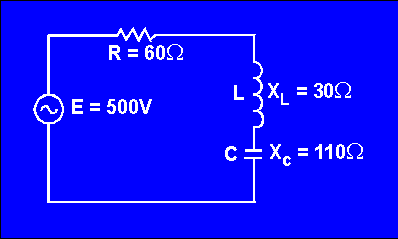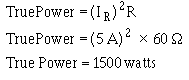voltage are in phase; that is, when the circuit is effectively resistive.">Custom SearchPOWER IN AC CIRCUITS You know that in a direct current circuit the power is equal to the voltage times the current, or P = E X I. If a voltage of 100 volts applied to a circuit produces a current of 10 amperes, the power is 1000 watts. This is also true in an ac circuit when the current and voltage are in phase; that is, when the circuit is effectively resistive. But, if the ac circuit contains reactance, the current will lead or lag the voltage by a certain amount (the phase angle). When the current is out of phase with the voltage, the power indicated by the product of the applied voltage and the total current gives only what is known as the APPARENT POWER. The TRUE POWER depends upon the phase angle between the current and voltage. The symbol for phase angle is q (Theta). When an alternating voltage is impressed across a capacitor, power is taken from the source and stored in the capacitor as the voltage increases from zero to its maximum value. Then, as the impressed voltage decreases from its maximum value to zero, the capacitor discharges and returns the power to the source. Likewise, as the current through an inductor increases from its zero value to its maximum value the field around the inductor builds up to a maximum, and when the current decreases from maximum to zero the field collapses and returns the power to the source. You can see therefore that no power is used up in either case, since the power alternately flows to and from the source. This power that is returned to the source by the reactive components in the circuit is called REACTIVE POWER. In a purely resistive circuit all of the power is consumed and none is returned to the source; in a purely reactive circuit no power is consumed and all of the power is returned to the source. It follows that in a circuit which contains both resistance and reactance there must be some power dissipated in the resistance as well as some returned to the source by the reactance. In figure 4-9 you can see the relationship between the voltage, the current, and the power in such a circuit. The part of the power curve which is shown below the horizontal reference line is the result of multiplying a positive instantaneous value of current by a negative instantaneous value of the voltage, or vice versa. As you know, the product obtained by multiplying a positive value by a negative value will be negative. Therefore the power at that instant must be considered as negative power. In other words, during this time the reactance was returning power to the source. Figure 4-9. - Instantaneous power when current and voltage are out of phase.The instantaneous power in the circuit is equal to the product of the applied voltage and current through the circuit. When the voltage and current are of the same polarity they are acting together and taking power from the source. When the polarities are unlike they are acting in opposition and power is being returned to the source. Briefly then, in an ac circuit which contains reactance as well as resistance, the apparent power is reduced by the power returned to the source, so that in such a circuit the net power, or true power, is always less than the apparent power. Calculating True Power in AC Circuits As mentioned before, the true power of a circuit is the power actually used in the circuit. This power, measured in watts, is the power associated with the total resistance in the circuit. To calculate true power, the voltage and current associated with the resistance must be used. Since the voltage drop across the resistance is equal to the resistance multiplied by the current through the resistance, true power can be calculated by the formula:For example, find the true power of the circuit shown in figure 4-10. Figure 4-10. - Example circuit for determining power.Since the current in a series circuit is the same in all parts of the circuit:Q.19 What is the true power in an ac circuit? Q.20 What is the unit of measurement of true power? Q.21 What is the formula for calculating true power?Integrated Publishing, Inc. - A (SDVOSB) Service Disabled Veteran Owned Small Business